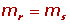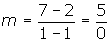Learn from home

The teachersThe slope is the inclination of a line with respect to the x-axis.

It is denoted by the letter m.Slope given two points:Slope given the angle:Slope given a vector of the line:Slope given the equation of the line:Two lines are parallel if their slopes are equal.Two lines are perpendicular if their slopes are the inverse of each other and their signs are opposite.The slope of the line through the points A = (2, 1) and B = (4, 7) is:The line passes through Points A = (1, 2) and B = (1, 7) and has no slope since division by 0 is undefined.If the angle between the line with the positive x-axis is acute, the slope is positive and grows as the angle increases.If the angle between the line with the positive x-axis is obtuse, the slope is negative and decreases as the angle increases.

Did you like the article?(1 votes, average: 5.00 out of 5)Loading...

Emma

I am passionate about travelling and currently live and work in Paris. I like to spend my time reading, gardening, running, learning languages and exploring new places.

Did you like
this resource?

Bravo!